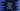# Python program to convert a list to string## Python program to convert a list to String :

In this example, we will learn how to_ convert a list to string. The list is one of the most commonly used datatype in python. List is used to storing a collection of different items. Python list is _mutable, ordered and it can also hold duplicate values. In python, list is created by placing all items inside a_ square bracket_ ([]). Items are separated by commas. The index of list item starts at 0, i.e. the index of the first element is 0, the index of the second element is 1 etc. We can access any element in a list by using its index. We can also delete or update an element in a list

The string is a collection of characters enclosed in quotes. We can use either single quotes or double quotes for creating a string. Even_ triple quotes_ can be used for creating a string in python.

In this tutorial, we will learn how to convert a python list to a string. The first thing that comes to our mind is to iterate through the list using a loop and concatenate each element to create the string. We can do that. But in this example, we will use the join method of String. join is defined as below :

```````str.join(iterable)
```````

The iterable passed to the join method should contain only string values. If it contains any non-string value, it will throw one TypeError. The value str is the separator string, i.e. the string that will be used to separate each element of the iterable. Let’s take a look into the program :

### Python program :

``````dummy_list = ["one","two","three","four","five","six"]

separator = ' '

result_string = separator.join(dummy_list)

print(result_string)``````

The example programs are available here.

### Output :

```````one two three four five six
```````

Let’s try by changing the separator to ’,’.

``````dummy_list = ["one","two","three","four","five","six"]

separator = ','

result_string = separator.join(dummy_list)

print(result_string)``````

Output is :

```````one,two,three,four,five,six
```````

Now, if we change one item of the list to an integer, it will produce the following output :